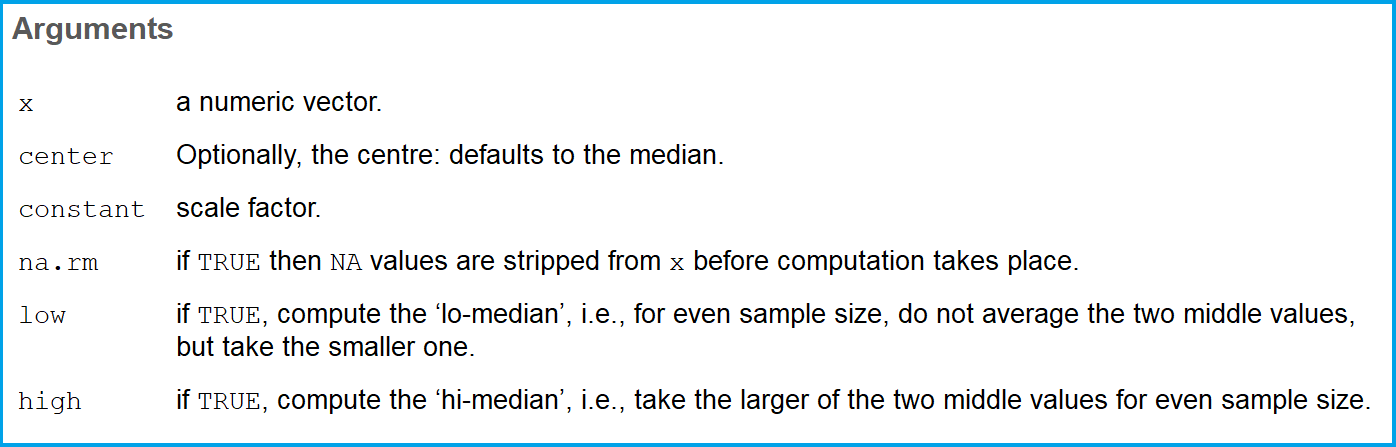# Median Absolute Deviation in R (Example) | mad Function Explained

In this article, I’ll explain how to compute the median absolute deviation in the R programming language.

The tutorial is mainly relying on the mad R function. The basic R syntax and the definition of the mad function are as follows:

`mad(x)`

The mad R function computes the median absolute deviation, i.e. the (lo-/hi-) median of the absolute deviations from the median.

In the following, I’ll show you an example code for the computation of the median absolute deviation in R.

Let’s jump right to it.

## Example: Median Absolute Deviation in R (mad Function)

In this example, I’m going to use the following numeric vector as example input:

`x <- c(3, 4, 1, 8, 2, 5, 2, 1)      # Create example vector`

Now, we can apply the mad R function in order to compute the median absolute deviation of this vector:

```mad(x)                              # Apply mad function in R
# 2.2239```

As you can see based on the output in your RStudio console, the MAD of our example data is 2.2239.

## Video & Further Resources

Below, you can find a video on the Statistics Globe YouTube channel where I describe the steps of this tutorial in expanded detail:

In the example above, I’ve shown you the basic application of the R mad function. However, there are several possibilities to modify the function. All options can be found in the R help documentation of mad:Figure 1: R Help Documentation of mad() Function.

In case you need another example for the application of the mad function in R, you may also have a look at the following YouTube video of Mr. Math Expert

Furthermore, you might be interested to read some of the other R tutorials of this website:

To summarize: This R programming tutorial explained how to calculate the median absolute deviation. If you have any questions, please let me know in the comments below.

Subscribe to the Statistics Globe Newsletter